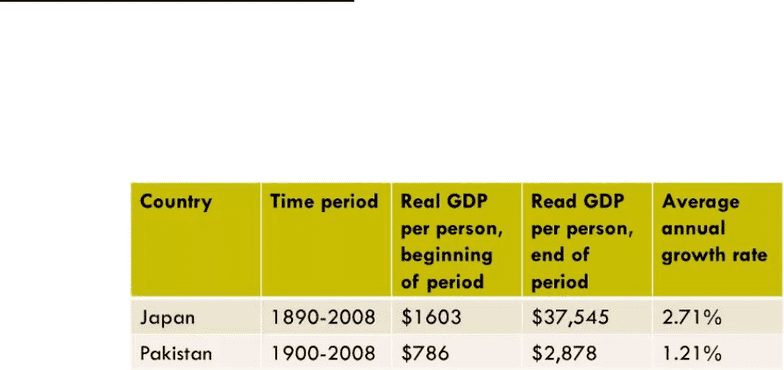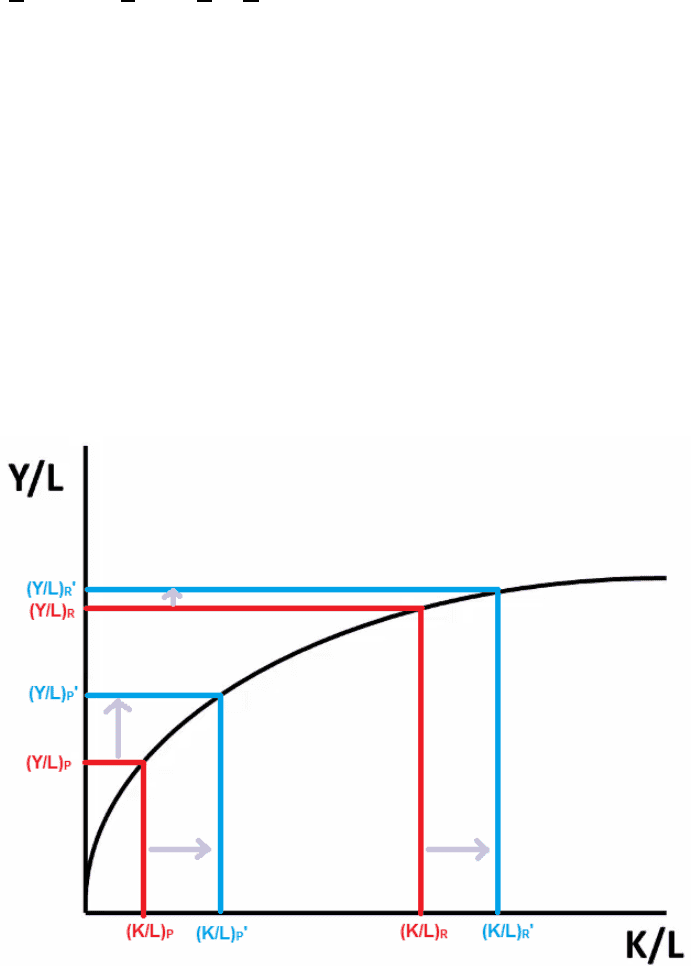# ECON 1BB3 Lecture Notes - Lecture 11: Aggregate Demand, Human Capital, Production Function

93 views6 pages
School
Department
CourseECON 1BB3: INTRODUCTORY MACROECONOMICS LECTURE 11 CHAPTER 7:
INTRODUCTION TO AGGREGATE SUPPLY AND AGGREGATE DEMAND
Ideas covered:
Economic Growth Facts and a Model
Policies to Enhance Growth
Aggregate Supply
Aggregate Demand
Economic Growth Facts and a Model
Growth: Small differences in growth rates can make a HUGE difference to a country’s
per capita GDP over time.
o Examples of two countries, Japan and Pakistan is shown below. At the beginning
of the time period, Japan’s real GDP per person was about twice as high as that
of Pakistan. Over the course of the next 100 years, Japan’s economy grew at an
average annual rate of 2.7% while Pakistan’s grew at 1.2%, a difference of only
1.5 percentage points. At the end of this long period, Japan’s real GDP per
person was about 13 times as high as Pakistan.
o If governments can find ways to increase their economic growth, the rate of real
GDP per person increasing over time then they can have significant impact on
the well-being of its citizens.
Productivity: the quantity of goods and services that a worker can produce in one hour.
o The higher the productivity, the higher the economic growth.
o Productivity is determined by:
Physical capital machinery equipment i.e. things that are used to
produces other goods and services.
Natural resources both renewable (such as forests) and non-renewable
(such as oil and minerals) resources.
Human capital
Technological knowledge
o Production function shows how we combine inputs to produce outputs.
Y = A . F (K, L, H, N) where, Y = output
A = technology
K = physical capital
L = labour
H = human capital
N = natural resources
o Output is the product technology and some function of the inputs.
o Technology allows the production of more output with the same combination of
inputs.
Returns to Scale:
o How does output respond when all inputs are increased by the same multiple?
Unlock document

This preview shows pages 1-2 of the document.
Unlock all 6 pages and 3 million more documents.Increasing returns to scale
Constant returns to scale doubling all inputs, doubles the output.
2Y = A . F(2K, 2L, 2H, 2N)
Decreasing returns to scale
o Assume that the production function exhibits constant returns to scale.
xY = A . F(xK, xL, xH, xN) where x = some multiple
Y = A . F( K , 1 , H , N ) by letting x = 1/L, Y/L = output per unit labour
L L L L K/L = physical capital per unit labour
H/L = human capital per unit labour
N/L = natural resources per unit labour
o The assumption allows us to look at the production function in aggregate terms
meaning what determines total output or in per worker terms meaning what
determines output per worker.
Marginal Product:
o Product = output
o Marginal = extra
o Marginal product is the output produced with one extra unit of an input.
o Diminishing marginal product: The additional output produced by adding the last
unit (19th unit) of labour is smaller than the additional output produced by adding
the second-last unit (18th unit) of labour.
o Assume that the production function exhibits diminishing marginal product.
o The assumption tells us about the shape of production function. By adding more
labour, the output produced increases at a decreasing rate.
Catch-up effect: Ceteris paribus, poor countries grow faster than rich countries.
o Suppose there exists a poor country with a (Y/L) lower than the (Y/L) for a rich
country and less (K/L) than the rich country. What happens is the same amount
of (K/L) is added to both countries? The horizontal shift in (K/L) is the same for
both countries. The increase in (Y/L) for the poor country is larger than the
increase in (Y/L) for the rich country.
Unlock document

This preview shows pages 1-2 of the document.
Unlock all 6 pages and 3 million more documents.

## Document Summary

Econ 1bb3: introductory macroeconomics lecture 11 chapter 7: Economic growth facts and a model. Growth: small differences in growth rates can make a huge difference to a country"s per capita gdp over time: examples of two countries, japan and pakistan is shown below. At the beginning of the time period, japan"s real gdp per person was about twice as high as that of pakistan. Over the course of the next 100 years, japan"s economy grew at an average annual rate of 2. 7% while pakistan"s grew at 1. 2%, a difference of only. At the end of this long period, japan"s real gdp per person was about 13 times as high as pakistan. If governments can find ways to increase their economic growth, the rate of real. Gdp per person increasing over time then they can have significant impact on the well-being of its citizens.

# Get access

\$10 USD/m
Billed \$120 USD annually
Homework Help
Class Notes
Textbook Notes